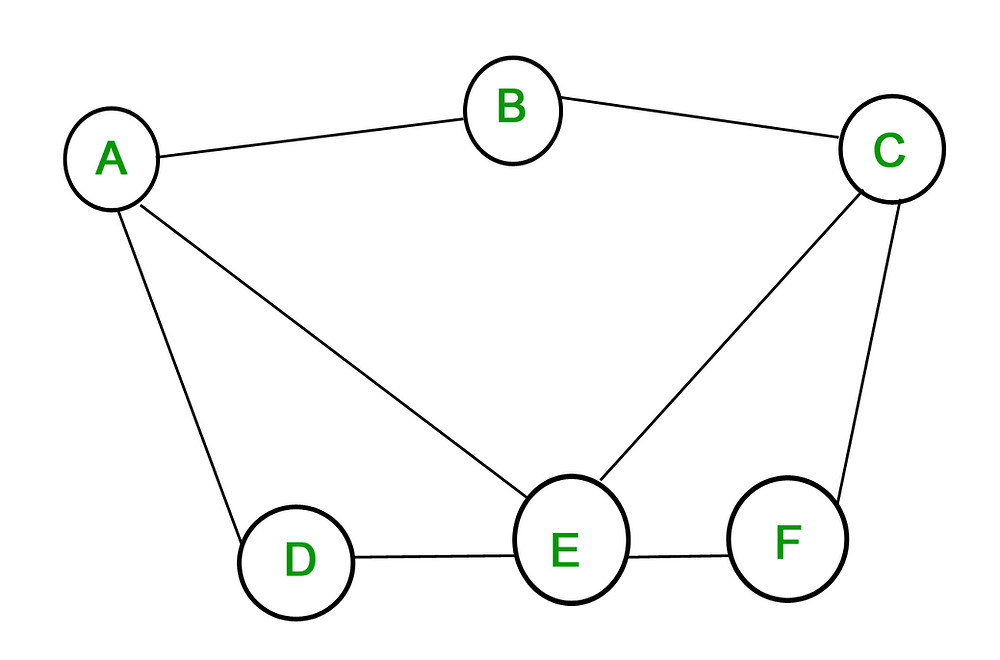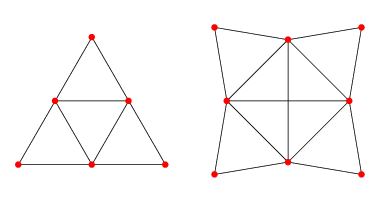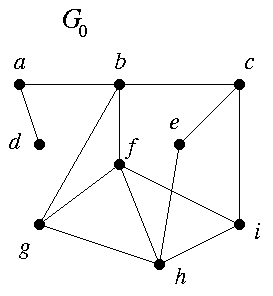Search

# Exploring the Four Color Theorem: Part 1

Updated: Dec 30, 2020

Hello everybody! Today's article is all about a classic problem: Given any map (think of a map of a continent or a map of the US), we wish to color the map so that any two regions sharing a border are different colors. What is the minimum number of colors we can use to guarantee we are always able to do this on any map?

For example, here's a way to color the US map in 6 colors:Courtesy of Safari Montage.

But can we do better? You might have already guessed the answer from the title: Any map (including the US map) can be colored in just four colors! The beauty of this problem, however, does not lie in merely coming up with an answer—but learning why it's true. If you know anything about the problem, you may have heard that it took supercomputers to solve it and that the proof for four colors is far from elegant. But what you might not know is you can completely understand the proofs for why you can always do it in six colors and why you can always do it in five, and both provide surprising insight into the essence of the subject. We'll walk down to the four color solution in this way: through the six and five color problems.

I will warn you that this does require a lot more formal mathematics (and a lot of definitions) than I usually use in my articles, but keep reading and persevering and things should fall into place for you—and it's a really exciting problem to grasp! My goal is to not just show you how to go about this problem, but to also introduce you to broad concepts like graph theory and induction that can help you across your explorations of math. It might make more sense if you already have a background in these topics, but I explain them all without any prior knowledge as I go on, so stick with it!

First, let's define a couple of rules to make sure we fully understand the stipulations of our problem:

1) Regions that share a boundary must be different colors. Think Georgia and Alabama.

2) Regions that don't share a boundary but do share a single point, like a corner, can be the same color! Think Colorado and Arizona.

Note: If this wasn't true, it would take any arbitrary number of colors to color a pizza shaped map, as every single one of the pizza slices shares the same point. This keeps our situation reasonablenow you can color a pizza in two or three colors.

3) Every region we color must be a continuous shape. Michigan, for example, is not one region but two. (It might be desirable to color Michigan the same color, but for the purposes of the math, we'll leave out this case, as it may force us to include more colors.)

Let's also define a couple of terms, even ones we already used, to make sure we know what we're talking about:

1) A map is, typically, a group of connected regions that lies on a plane (meaning it's 2D and can fit on a sheet of paper).

2) A region is a section of a map that is one continuous shape, like a state on the US map. It will be colored one uniform color.

2) A coloring is one possible way of coloring the entire map. Here, we want colorings that meet the stipulations specified above.

4) A map is n-colorable if it can be colored (in the way specified above) with n colors.

For example, because of the coloring we showed of the US map above, we can say that the US map is 6-colorable.

Here's a coloring that requires 5 colors (ignore Alaska):Courtesy of Computational Complexity.

We can now say that the US map is 5-colorable.

And finally, here's a coloring that only requires 4 colors:Courtesy of Geology.com.

We can now say that the US map is 4-colorable.

Our claim: Every map is 4-colorable.

Note that we are in no way finding out how to come up with the particular coloring that only requires four colors—and there may be several, even lots, of possible colorings! This is an existence problem, meaning our main goal is to prove that something is always possible, that an appropriate coloring always exists, not to show how to obtain that particular coloring.

This article has two parts. This week, we're beginning to work our way down to four colors, introducing the concepts of graph theory and induction to explain the Six Color Theorem, and next week, we'll be looking at the Five Color Theorem and the Four Color Theorem and we'll also be examining an interesting statement set aside from this week.

So let's get started with the Six Color Theorem (i.e. Every map is 6-colorable)!

To make this easier to work with, I'm going to define something called a graph. This problem actually falls in a field called graph theory—which I'd love to explore more in detail (and give you more of an introduction to) in more articles! For now, we're only going to go over what we immediately need to know.

In graph theory, a graph is a set of edges and vertices (nope, it's not the type with coordinate axes here!)

It can look something like this:Courtesy of GeeksForGeeks

Here, A, B, C, D, E, and F are vertices and the connections between them are called edges.

Here's another example:Courtesy of Wolfram MathWorld.

Vertices in graphs often have labels. Here, ours are going to have colors!

At this moment, I'm also going to define a term called degree. A vertex has degree n if there are n edges coming from it. For example, in the first graph, vertex A has degree 3, vertex B has degree 2, and vertex E has degree 4.

We're going to turn our maps into graphs, while keeping the essential properties of our maps. We're going to draw a graph from our map by turning every region into a vertex and connecting any two vertices whose corresponding regions share a boundary (more than just a single point) with an edge.

Here's part of a map of Europe, transformed from a map to a graph. All this transformation requires is drawing a vertex at each country and connecting where appropriate:Courtesy of the Princeton Math Department and Perry-Castaneda ˜ Library Map Collection at the University of Texas

We no longer need the map, so we can take the graph off and stretch it out a little bit to make it more readable (the exact shape is not important, only the number of vertices and the appropriate connections between them):Courtesy of Princeton Math Department

The problem becomes: What is the minimum number of colors we can use to color every graph that is planar (meaning any graph with NO edge crossings—all graphs from maps meet this requirement) such that any two vertices that are directly connected by an edge are two different colors?

This question is equivalent to the problem we stated for maps, and if we solve it, we solve the problem for maps as well. A correct coloring of the graph corresponds directly to a correct coloring of the map.

Look! Here's our graph from our map in four colors!Courtesy of Princeton Math Department

Ok, now to the Six Color Theorem!

We want to prove that every map is 6-colorable, so we prove that every planar graph is 6-colorable.

We're going to use a lemma (a smaller theorem) to build off of to prove this statement.

Lemma: Every planar graph G must contain a vertex of degree 5.

(To keep this article moving forward, I won't prove this here, but I want to keep this series comprehensive, so it will be in next week's article! Stay tuned!)

This lemma means that every vertex in a graph cannot have a degree of 6 or more, and at least one vertex has a degree of 5 or less (and usually many more vertices meet this condition as well!)

For example, in the graph above, Switzerland could be the vertex of degree 5 or less, or we could choose Spain or even Vatican City. What matters to the proof below is that we choose one vertex that meets that condition, not which one we choose.

We're going to use a strategy called induction to complete this proof. Here is an explanation of induction.

In induction, we prove that the first case of a theorem is true and we use the fact the first case is true to prove the second case is true and the second case to prove the third case and so on, until we know that every case of the theorem is true. Let me break it down.

Say we want to prove 1+2+3+4+5+...+n = n(n+1)/2 for every positive integer n. Here our cases are the infinite integer values of n.

In induction, we first prove the first case is true. This is called the base case.

When n = 1, we need 1 to equal 1(1+1)/2 = 1(2)/2 = 1, so the base case is indeed true.

Then, we need to prove that if the nth case is true, the (n+1)th case is also true. This is called the inductive step. (Notice that when we prove this is true, we prove that the first case being true implies the second case is true, and the second case being true implies the third case is true and so on, all the way up the positive integers, so every case is true.)

To complete the inductive step, we start with an inductive hypothesis. We assume that the nth case is already proven true.

Here, we assume 1+2+3+4+5+...+n equals n(n+1)/2 for some n.

Then, we use that assumption to show that the nth case does indeed imply the (n+1)th case is true.

Here, this looks like the following:

1+2+3+4+5+...+n+(n+1) = n(n+1)/2 + (n+1)

(We use the inductive hypothesis to sub in n(n+1)/2 for 1+2+3+4...+n)

Then, we can simplify this to give:

(n(n+1) + 2(n+1))/2 = (n^2 + 3n + 2)/2 = (n+1)(n+2)/2, which was exactly the result we wanted. We wanted 1+2+3+4+5...+n+(n+1) to equal (n+1)(n+1+1)/2, which is simply plugging in the value n+1 for n.

This completes the inductive step and it also completes the proof, as the base case and the inductive step together show that 1+2+3+4+5...+n = n(n+1)/2 for all values of n.

Think about induction as proving that a chain of dominoes will fall. We first prove that the first domino will fall, then we prove that every domino falling will also cause the next one to fall. Together, these two steps prove that the entire row of dominoes will fall and the theorem will be true for every domino and every case.

For induction to work, you need to have cases that can be numbered: a clear first case, a clear second case, a clear third case, and so on. Here, our cases were indexed by the integer values of n.

I encourage you to watch this video before you continue if you need more time to grasp induction:

Ok, let's apply what we just learned to the Six Color Theorem: Every planar graph is 6-colorable.

We're going to be completing induction by the number of vertices, showing that we can add a vertex to a graph and it will still remain 6-colorable. Our goal is to prove that every graph with V vertices is 6-colorable for any value of V, so here, our cases are indexed by the integer values of V.

For the base case, note that the statement is clearly true for V ≤ 6. We have no problem coloring all the vertices of a graph different colors if we have more colors than we have vertices. By induction, we can show it is true for V=7,8,9,... as well.

Now, for the inductive step, we start out by assuming that the statement is true for V = k (this is our inductive hypothesis). We want to show that the statement being true for V = k implies it's also true for V = k+1.

Say G is a graph with k+1 vertices. By our lemma, G has at least one vertex with degree ≤ 5.

Let's say that vertex looks something like this:The 5 (or less) vertices connected to the central vertex may have any number of other connections and may connect to each other on the outside of the graph, like the vertices around f below do. We've simply isolated the vertex in our drawing to make it easier to narrow in on exactly what we want to see.Courtesy of Binghamton University Math Department.

Then, we can assume that the planar graph without the central vertex is 6-colorable, since it has k vertices, and we assumed by our inductive hypothesis that any graph that has k vertices is 6-colorable.And guess what? If this graph is 6-colorable, then so is G! The central vertex is connected to 5 or less vertices and hence, 5 or less colors, so all we need to do is color the central vertex a sixth color that is not represented!

This completes the inductive step, and hence, the proof.

Since we know a graph with 6 or less vertices is always 6-colorable and that each time every graph of k vertices is 6-colorable, every graph of k+1 vertices is as well (as there is always at least one vertex of degree 5 or less to consider), we know that every single graph with any number of vertices is 6-colorable! Hence, every possible map is 6-colorable as well!

Sometimes induction seems a little bit like magic. We've come at this problem indirectly rather than proving exactly how we would color each graph. But, if you think about it, this makes sense. We basically can construct our graph in the way we explained this by induction, as this proves that every time we add a vertex to a graph it is still 6-colorable, and proving that the process of building a graph doesn't change 6-colorability also proves any graph you can build is 6-colorable.